# Graphing

With over 100 built-in graph types, Origin makes it easy to create and customize publication-quality graphs. You can simply start with a built-in graph template and then customize every element of your graph to suit your needs. Easily add additional axes, as well as multiple panels/layers to your graph page. Save your settings as a custom template for repeat use. In addition, you can do batch plotting with other dataset.

## Column, Bar & Pie Graphs

Column, Bar and Pie Charts are widely used across all fields, for their simplicity and ease of interpretation. Origin supports:

• Basic, stacked, grouped Column & Bar chart
• Floating Column & Bar chart
• 3D Column chart
• 2D pie chart
• 3D pie chart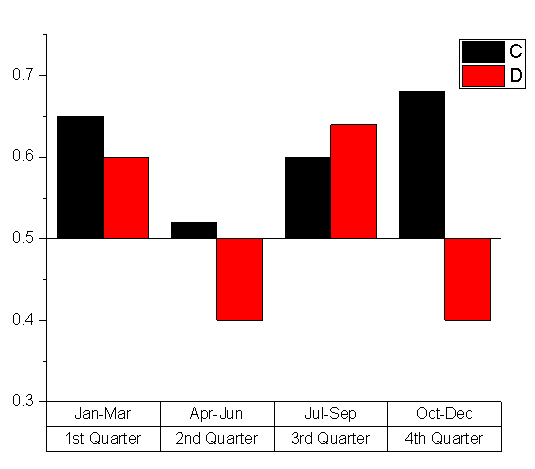A column plot starting from Non-zero Value. Customization options for axes include positioning bottom X axis at a non-zero value, turning on the top axis and moving it to the bottom to show tick labels at bottom. Tick labels can be shown as a second row table.Grouped column plot with asterisk bracket to show significant differences. Options for customization include flexible spacing between subgroups, placement of asterisk brackets to highlight comparisons and patterns for each column.A 3D bar plot on a flattened surface, showing the population distribution of the United States. This graph is created by flattening a 3D surface of US Map and then plotting the city population as 3D bars on it. The surface is set transparent. Add a 5-column plot to the right as a creative Legend.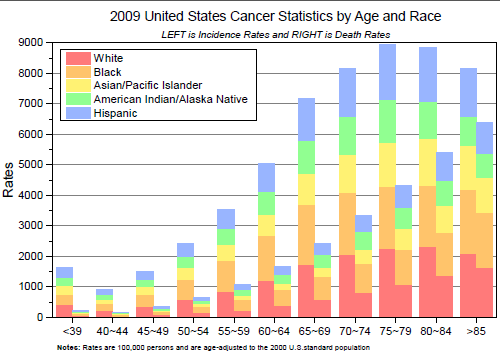This grouped stacked column plot is created by plotting columns into subgroups of age range, adjusting spacing between and within subgroups, and then stacking death rates of different Races cumulatively.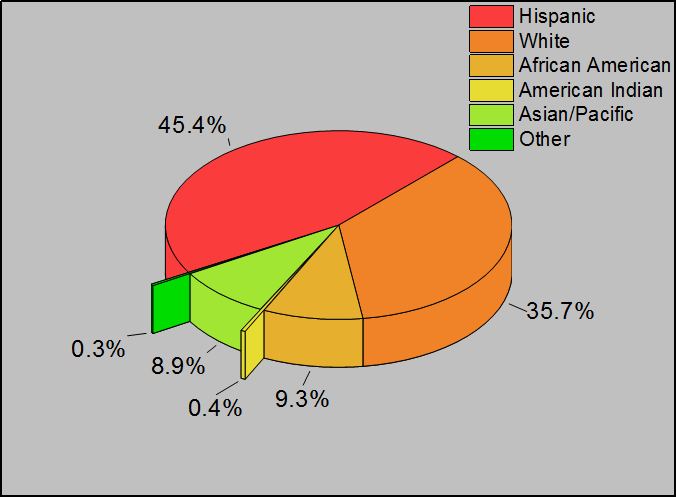A Pie chart with indexed colors, 3D effect, and exploding sections for clarity of minor constituents. Additional options include controlling the thickness of the pie slice, displacement, view angle, size and rotation of the chart and leader lines of the labels.

## Line & Symbol Graphs

Origin supports:

• Line plot
• Row-wise Line Series Plot
• Scatter plot (with XY Error bar, Column Scatter, Drop line, Color Map, Size Map)
• Line + Symbol plot
• Area plot
• Area
• Stacked Area
• Fill AreaFill area between two curves. Control of the fill colors of areas above and below the reference curve allows for the use of different colors if desired.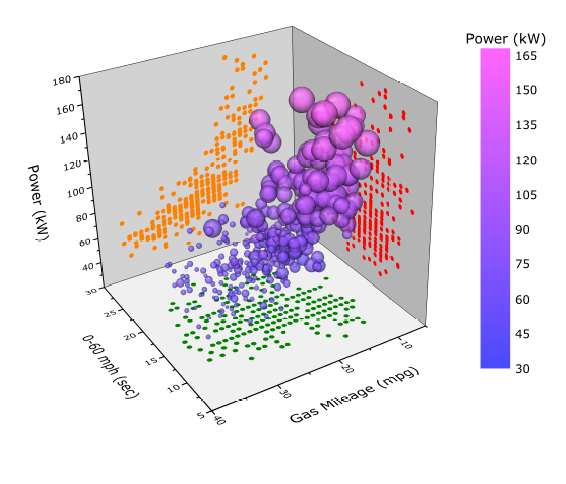3D Color-Mapped Scatter Plot. Customization options include linking symbol size to another column (Engine Displacement) and enabling projections on all three axes planes.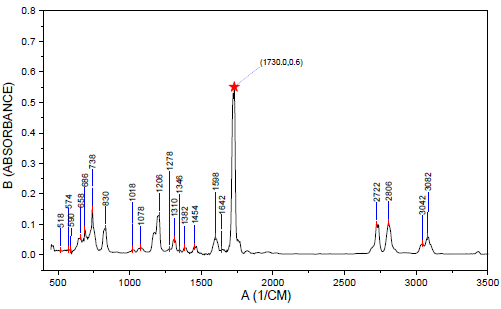Smart labeling of peaks with a vertical offset and a customized special point. Label customization options include offsetting the labels, and smart re-positioning to avoid overlapping, Creating a special point allows for different formatting and positioning.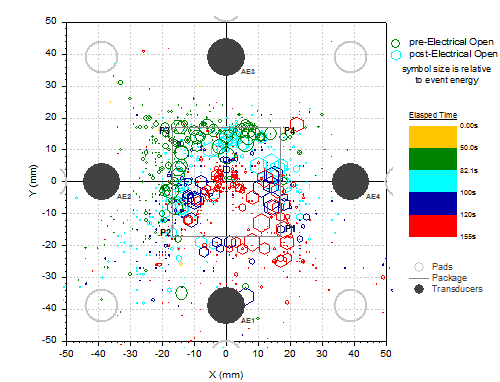Scatter Plot of Acoustic Emission Detection. Symbols are positioned to show the relative location the events while shapes differentiate pre or post-Electrical Open. Size and color are mapped to the event energy and event time respectively.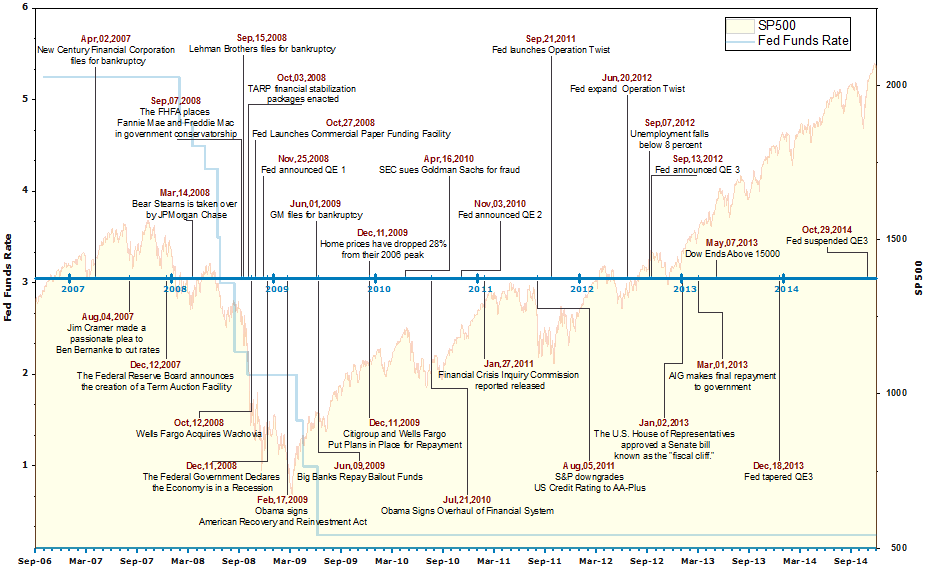TimeLine for Financial Crisis. This graph consists of 3 layers; the first two share the bottom time axis while the third contains a middle time axis linked to the bottom axis by custom linking. The two time axes have the same range but different increments. The timeline events on the middle axis are created by using scatter plots and timeline labels are connected to the corresponding event by flexible leader lines.Scatter plot depicting the isotope signature of N sources found in IAB iron meteorites. The graph is created by plotting the points with X and Y error bars while indexing symbol colors to distinguish between etched and un-etched samples. The bounding triangle is plotted by a dotted line plot. An additional scatter plot with labels is then added on the top line to show the mixing ratios for the two proposed sources.

## Multi-Axis & Multi-Panel Graphs

To present multiple datasets in the same graph window, Origin provides:

• Multi-axis graph
• Multi-panel graphYBCO superconductor growth study using 3 sets of axes including Disposition Pressure, Annealing Temperature, and Delta Temperature over time. Additional customization includes cubic B-spline connection-type for the Delta Temperature line + symbol plot. Error bars (in both directions) are included for the Annealing Temperature scatter plot.Multi-panel plot shows experimental x-ray emission (XES) and absorption (XAS) spectra. The graph contains seven layers. The upper and lower-right layers are grouped XES and XAS line plots, one with an inset plot. The four layers on the lower-left are X-axis-linked color fill contours. All layers can be resized and repositioned flexibly.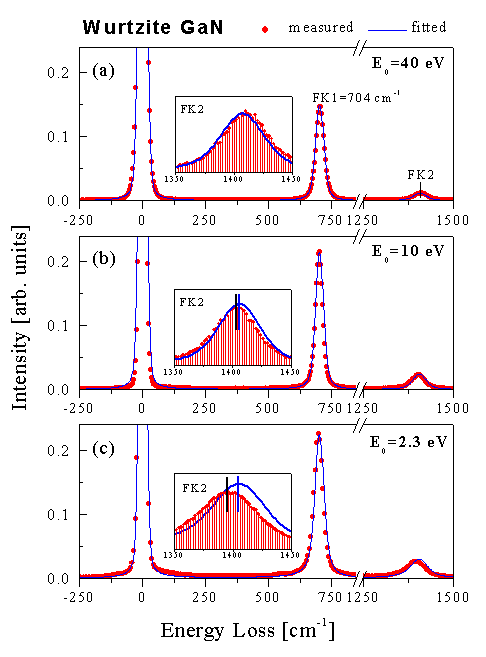Multi-panel graph presenting high-resolution electron energy-loss spectra from a GaN surface. The three main panels present measured data (red filled circles) and calculations (solid blue line), with linked X axes and axis breaks added. The inset plots in the three panels display a section of the data (red fill circles with vertical drop-lines) for emphasis.

## Ternary & Piper Graphs

Ternary and Piper diagrams are two specialized graph types with applications primarily in the geosciences and civil engineering. Origin supports:

• 2D Ternary (with Scatter or Contour)
• 3D Ternary (with Scatter or Colormap Surface)
• Piper chartZooming into a Section of a Ternary Plot by Setting Axis Scales. This graph is made by adjusting the plot to a region of parallelogram shape.A 3D ternary surface with projection. The 3D ternary surface plot is created from a XYZZ data, while the projection at the bottom is the same surface flattened with only color-mapping contour line shown.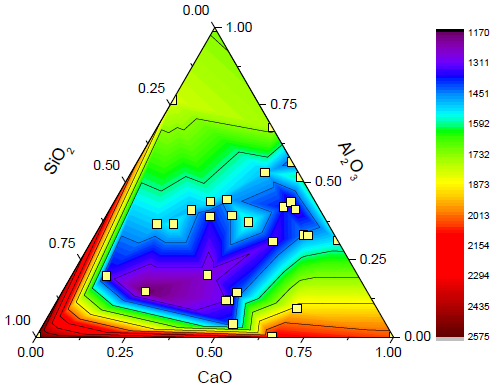Ternary contour plot with data point overlay. The ternary contour is created from a XYZZ data, where the first XYZ is the ternary coordinates normalized between 0 and 1 while the second Z is used for color-mapping. The same first XYZ is also added as a scatter plot on top.Ternary diagram of Soil Analysis Packing Fraction. Each line in this graph is created from a XYZ dataset stored in a separate worksheet. Customization options include labeling line plots with their corresponding packing fraction values and connecting data point with B-Spline line.Piper diagram with TDS and point by point legend. Piper Diagrams contain 3 linked layers with normalized data. Customization options include adjusting the gap between ternary and rhomb, indexing symbol size and color, adding sample ID as label, and updating legend showing data location information point by point.

## Polar Graphs

Polar graphs are relevant to any phenomena characterized by its direction and distance from a fixed point, for example, temperature distribution in Earth's Polar Regions. Polar graphs are also useful for intuitive visualization of multivariate data. Origin features easy-to-use templates to display data and functions in polar coordinates, including radar and windrose diagrams.Polar chart with log scale. Customization options include setting log scale for radial axis and color-mapping the line by the date or any other variable.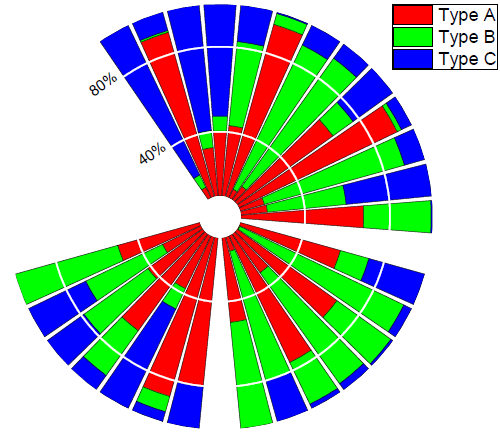Stacked Polar Column Plot. Customization options include stacking bars of different "types", showing only one radial axis at 120 degrees, and setting the radial axis to start from a non-zero value.Fibonacci Sequences. The graph can be created by generating data with LabTalk scripts, plotting a theta(X) r(Y) polar graph and then mapping symbol size and color to the r and theta values.Polar contour with custom orientation and contour line. The polar contour plot is generated directly from a matrix data. Customizations for axes include setting angular axis orientation to clockwise from -30 degrees and attaching the radial axis to the end angle.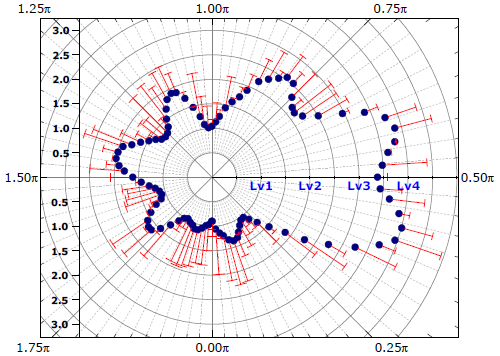Cropped polar chart with custom orientation and radian scale. Customization options for axes include changing the range and orientation of the angular axis, adding more radial axes and customizing their orientations, ticks, and labels, and cropping the graph to show only a portion of the data.Two polar contour plots overlaid with one polar chart. This graph is created by merging three graphs, two polar contours (surface temperature as a color-filled contour and sea level pressure as green contour lines) and one polar chart (the coastline of the Northern Hemisphere) in two layers. Transparency is set to both the color-filled contour and the contour lines to make the bottom polar chart visible.

## Waterfall Graphs

Origin's Waterfall graphs are ideal for comparing variations between multiple datasets created under similar conditions. The graph has a three-dimensional effect, enabling you to see variations in the Y or Z-direction.

• 2D Waterfall
• 3D Waterfall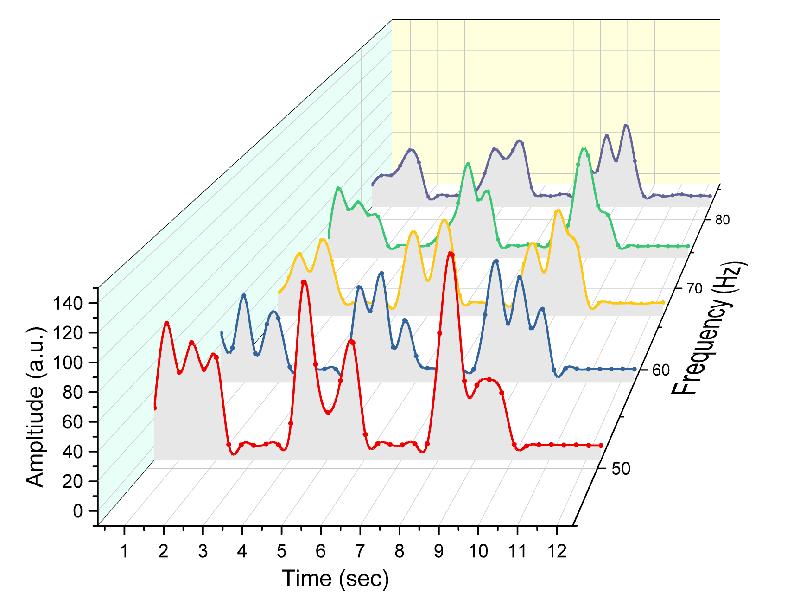3D Waterfall supports Connect Control which enables watefall to have smooth lines even though data points are few.Waterfall with a single traced value highlighted with different color. The line series are ungrouped so that the desired trace can be set to a different color.

## Contour & Heatmap

Origin's contour graph can be created from both XYZ worksheet data and matrix data. It includes the ability to apply a color fill and/or a fill pattern to the contours, display contour lines (equipotential lines) and contour labels, as well as adjust contour levels. A color-scale object can be included with the contour plot to serve as a legend. XY data of contour line can be extracted.Polar contour plot of semiconductor wafer thickness measurement as a function of the wafer radius and angle. Polar contour plots can be created from data organized as "theta, radius, Z" or as "radius, theta, Z".Color fill contour depicting the global mean distribution of seawater conductivity. The conductivity data stored in a matrix is plotted as a color fill contour, with contour lines hidden. The missing values in the matrix where continents are located, are colored white. A second dataset containing continent boundaries is plotted as an overlay line graph.Contour plot depicting vertical wind velocities as a function of time and height, overlaid with a vector plot depicting wind speed and direction. The graph was created by merging a color-fill contour of vertical wind velocities data, and a vector plot of wind speed and direction data (in the form of X, Y, Angle, and Magnitude). The axes of both plots were set to be identical for the overlay.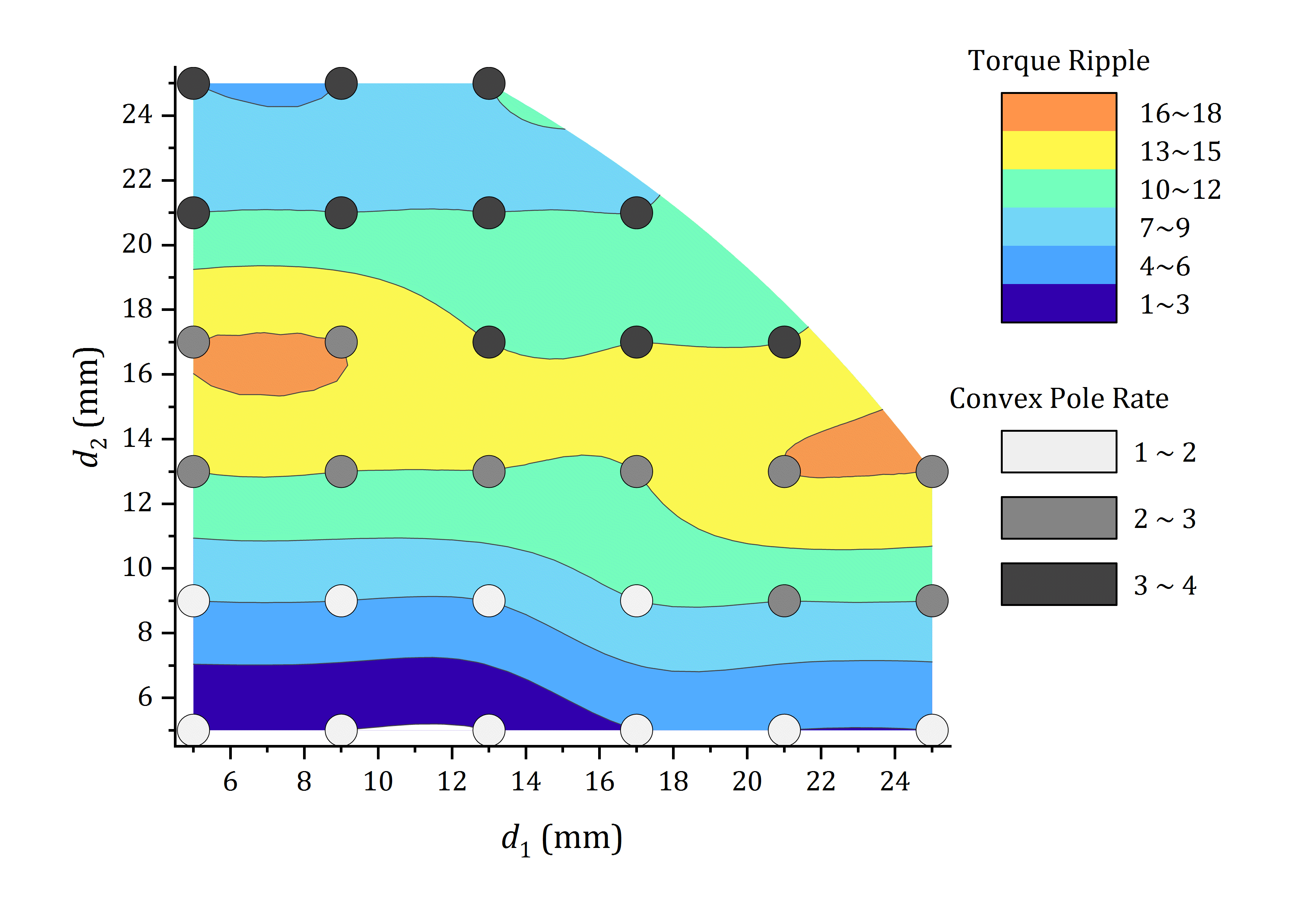Contour created from XYZ columns where Z is categorical (text). A scatter plot with color map was also added to the layer.XYZ contour plot with custom boundary depicting 30-year mean temperature for continental United States for the month of January. The contour plot was created directly from XYZ data columns. Customization options for contour include specifying custom boundary from another dataset, changing levels and color palette, hiding minor level contour lines, and customizing individual contour lines at a specific level.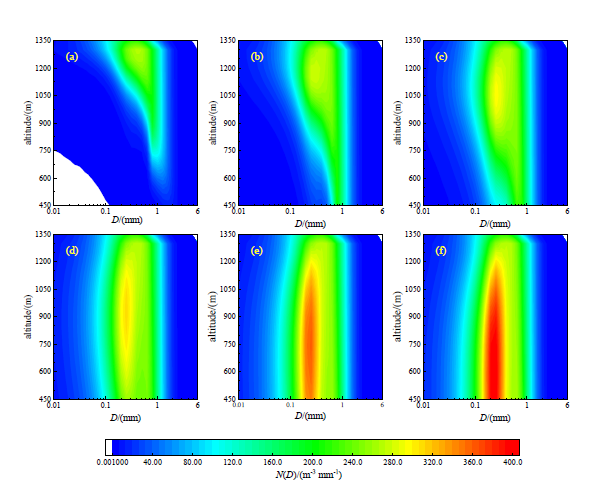This graph is merged from 6 contour plots. The graphs simulate the change of raindrop spectra with height and time. Figures a-f respectively show the simulation results of 2, 4, 6, 10, 40, 60 min.Create Heatmap plot from XYZ or XY data columns. Option to specify custom bin settings for X and Y. Generate output with counts, sum, min, max, mean, median or percent frequency.

## Vector Plots

A vector graph is a multidimensional graph used in industries such as meteorology, aviation, and construction that illustrates flow patterns (e.g. of wind, water, magnetic field), and represents both direction and magnitude at each point. Origin provides:

• 2D Vector graphs
• 3D Vector graphsThis vector plot was created from data organized as X,Y, angle, and magnitude. The vector color was mapped to the magnitude values, illustrating the effect on river water flow around differently shaped pylons. The two pylons were drawn using circle object and a fill-area plot, added as a second layer.An overlay plot depicting worldwide Ocean currents. The continents were plotted as a fill area graph, with the setting "inclusive broken by missing values" selected. The warm and cool currents were graphed as vector plots with labels(data organized as X, Y, angle, and magnitude). The two graphs were then merged and superimposed.

## 3D GraphsOrigin supports free transforms of 3D plots. Some transforms can be done in real time, e.g. rotation, stretching or skewing. Origin provides:

• 3D Symbol/Trajectory/Vector Plot
• 3D Basic and Stacked Bar Plot
• 3D Surface Plot
• 3D Function Plot
• 3D Ribbons/Walls Plot
• Tetrahedral Plot
• 3D Waterfall
• 3D TernaryThis plot shows a 3D scatter plot with x, y, z errors, and projections on three axis planes. The 3D scatter symbol is colormapped to another data column (population density). Symbols and error bars in each projection can be customized independently.This is a 3D Stacked Bars plot, with bar shape set to Cylinder. The plot tracks emissions of three classes of greenhouse gases, in the countries of France, Germany, Canada and Japan, over the period from 1990 to 2010.Colormap surface with side walls, showing the topology of eastern California. The surface is overlaid by a 3D scatter plot with label to highlight two specific locations. Lines connecting data points and labels can be rotated along with the frame, and were added with LabTalk script. Lighting effect was also turned on.Multiple surfaces stacked in a single layer. The graph was created by plotting the same matrix data in four different styles, and offsetting them in Z direction. The four surfaces from top to bottom are wire frame, flattened contour line plot, color filled surface with lighting effect, and flattened color filled contour plot.This is an XYY 3D 100% Stacked Bars plot comparing monthly regional sales, for two makes of automobile (BMW and Ferrari).A surface plot created from XYZ data where the color map was based on a 4th data column. A custom XY boundary has also been applied to the plot.A combination of 3D bar and 3D scatter plot depicting home price index and unemployment rate. Customization options include color mapping both plots based on Z values, adding labels to 3D scatter points, hiding YZ and ZX planes and moving XY plane to the center.

## Grouped Data PlotUsing grouped data, you can easily create multi-panelled graphs in Origin with a single click on the plot menu

• Cluster Plot (Line, Scatter and Column)
• Grouped Box Plot
• Grouped Column Plot
• Various Types of Trellis Plots

• Scatter
• Line and Symbol
• Bridge Chart
• Box
• Double - Y

• Column/Bar
• Stacked Column/ Bar
• 100% Stacked Column/ Bars
• Floating Bar

Flexible configuration and customization options include

• Control the style increments between or within the groups
• Customize the gap between, or within the groups.
• Add Gap between panels
• Wrap panels
• Overlap panels
• Uniform or Independent X/Y Scale
• By Drag-and-Drop Column Assignments (with Pre-installed App - Graph Maker)The user can easily create multi-panel graphs from grouped data with the Trellis plot.Multi-layer Cluster Plot with option for independent X and Y scales. Easily change formatting of plots and other attributes in all layers by editing properties in one layer.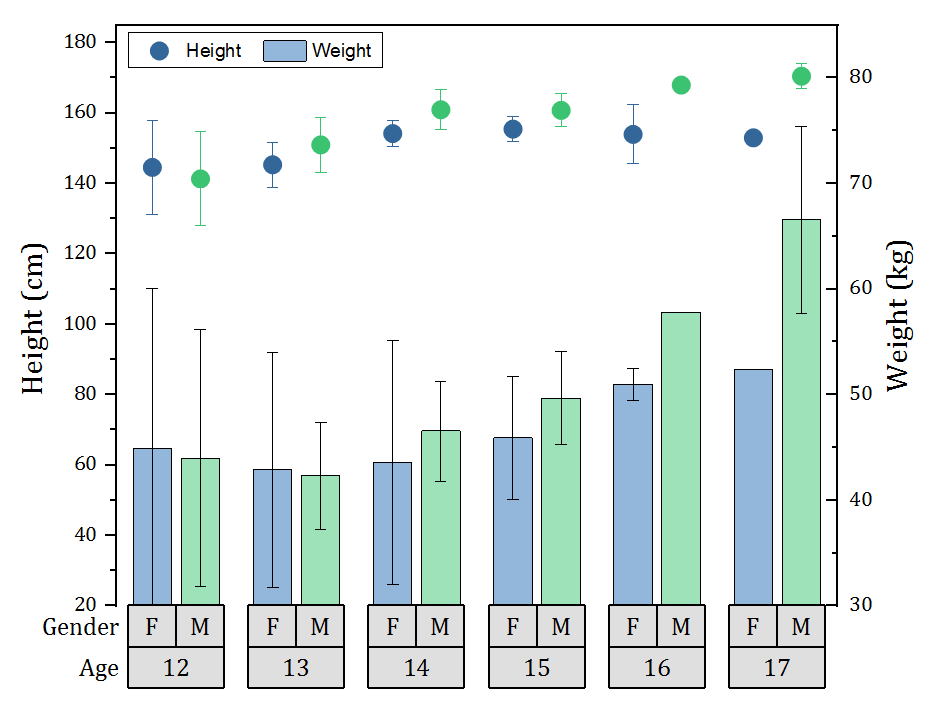Grouped plots now support scatter plot with subgroup spacing. Scatter can then be combined with other plot types such as the column plot in this example.Double-Y Trellis plot with two independent Y axes, each with its own scale settings.Grouped box chart with gap between subgroups. The graph was created from indexed data with two group levels. Options for customization include flexible spacing between and within subgroups, setting axis tick label as a table above or below the graph layer to display relevant grouping information, connecting mean points, data points or other percentiles and multiple box styles including column scatter, statistics bars and interval plots.This is an example of a trellis column plot with error bars. In the trellis plot, the horizontal panels are defined by two grouping variables, treatment (Memantine v.s. Saline) and genotype (Control v.s. Ts65Dn), and the vertical panels by one grouping variable, behavior (C/S v.s. S/C).The example shows a trellis plot with the Overlap Panels option enabled. Two variables, Location and Treatment, are used to define the horizontal panels. This results in a four-panel horizontal array. By enabling the Overlap Panels option, we combine four panels into one while preserving the grouping information. Note that plot symbol color is indexed to the four Location x Treatment combinations and symbol shape is indexed to Year, as shown in the plot legends.

## Statistical Graphs

Origin upports many types of Statistical graph:

• Box Chart, Grouped Box Chart
• Histogram, Stacked Histograms
• Marginal Box/ Histogram Chart
• Histogram + Probabilities Plot
• Rug Plot
• Violin Plot
• Scatter Matrix Plot
• Bridge Charts
• Probability & Q-Q Plot
• 2D Kernel Density Plot
• Parallel Plot
• QC(x bar R) Chart
• Pareto Chart
• Statistics Bars
• Interval PlotHalf Violin plot displaying density distribution and data points. Visit this blog page to view more Violin plots.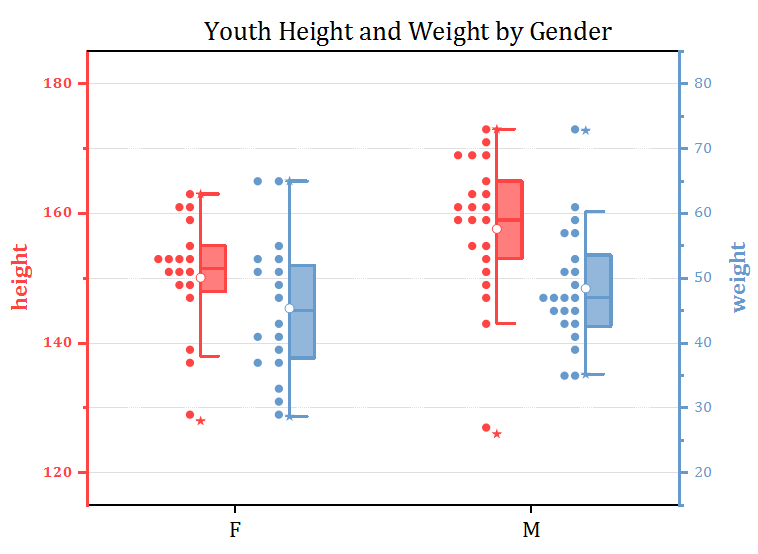The Double-Y Half Box plot displaying box and data points, The data points are aligned in bins to show the distribution. The graph has two independent Y axes, each with its own scale settings.Histogram and probabilities chart: The histogram in Layer 1 provides the center, spread, and skewness of the data, while the probability plot in Layer 2 indicates whether the data follows a normal distribution.2D Kernel Density plot showing the relationship between gasoline price and houses for sale. The 2D Kernel Density plot is a smoothed color density representation of scatter plot, based on kernel density estimation. Customization options include the calculation method and flexible color-mapping with palettes.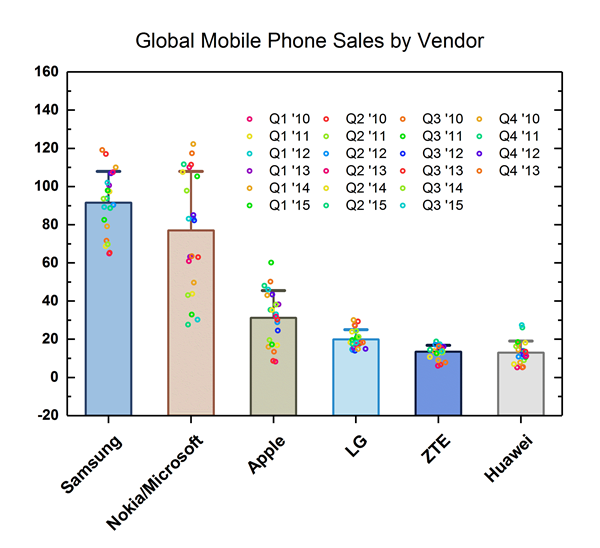This graph displays a bar chart with data points overlapped. The bars represent the means of the datasets. Bars can be set to show other quantities (sum, median, max, etc.), using controls on the Box tab of the Plot Details dialog.A stacked Bridge Chart displaying Value and Proceeds. Additional options include setting multiple Total columns, showing connect line, connecting by subset ect.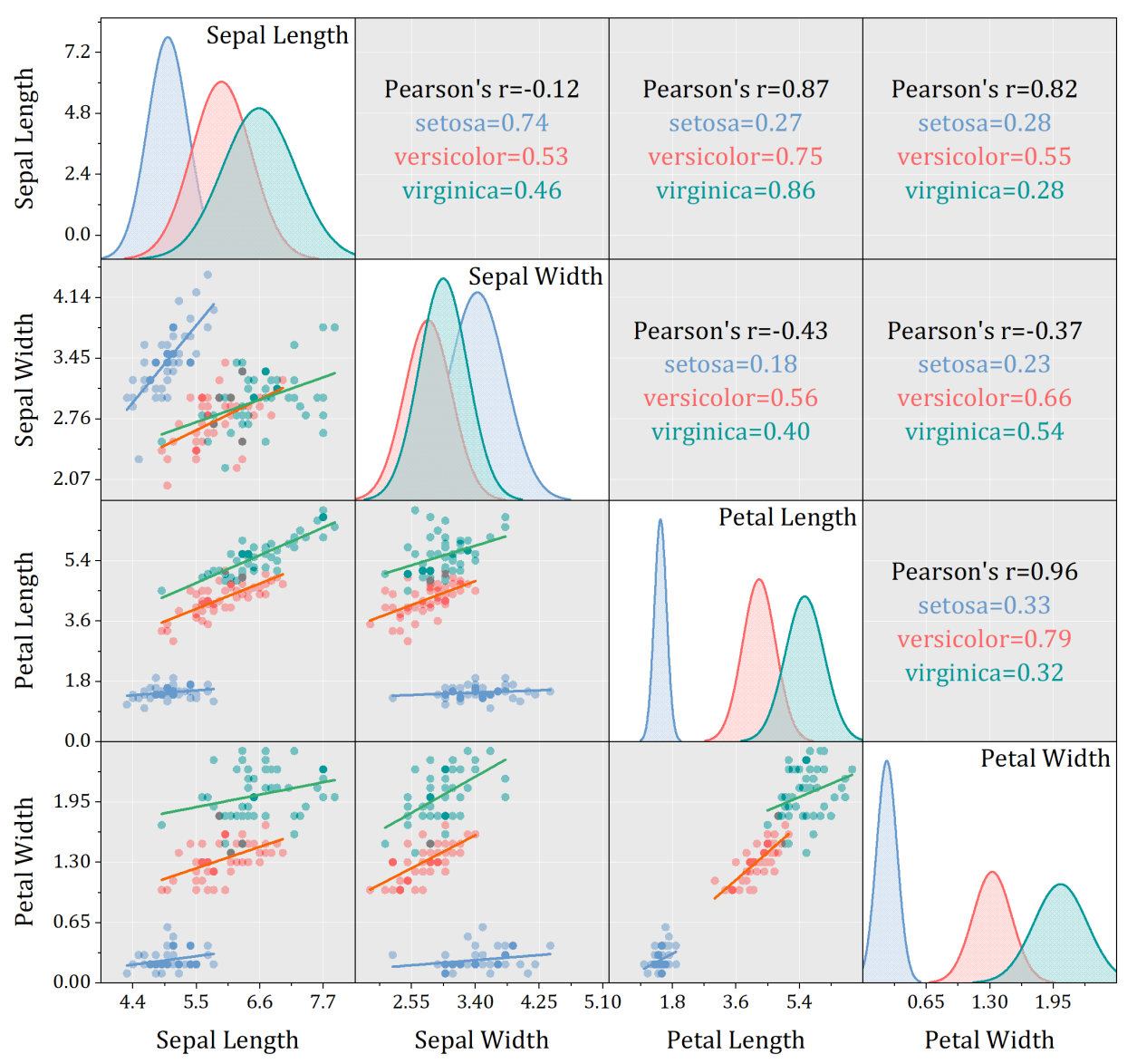Scatter matrix with histogram in diagonal cells. A scatter matrix consists of several pair-wise scatter plots of variables presented in a matrix format. It can be used to determine whether the variables are correlated and whether the correlation is positive or negative. Customization options for scatter matrix include changing the background color of the entire window and scatter plot layers, coloring mapping scatter points by group, and hiding scatter plots on the upper/lower triangle of the diagonal.Pareto chart depicting food defects in a sampling test. Pareto chart consists of bars and a line plot. The individual values are displayed in descending order by bars, while the cumulative percentage of the total number of occurrences is represented ascending monotonously by the line. Pareto charts can be created from raw data and binned data. Customization options include adding labels for both bar chat and line plot, coloring bar with a custom color list, and fading bar color from top to bottom.

## Specialized Graphs

Origin upports many types of Specialized graph:

• Wind Rose (Binned/Raw Data)
• Radar/Spider Plots
• Smith Charts
• Zoom Graph
• Stock GraphsWindrose graph displaying wind speed and direction. The length of each "arm" is proportional to the fractional frequency at which wind was observed from that direction, while different colors on each "arm" indicate the wind speed. Windrose graphs can be created using both binned data and raw data and customization of the direction tick labels is supported.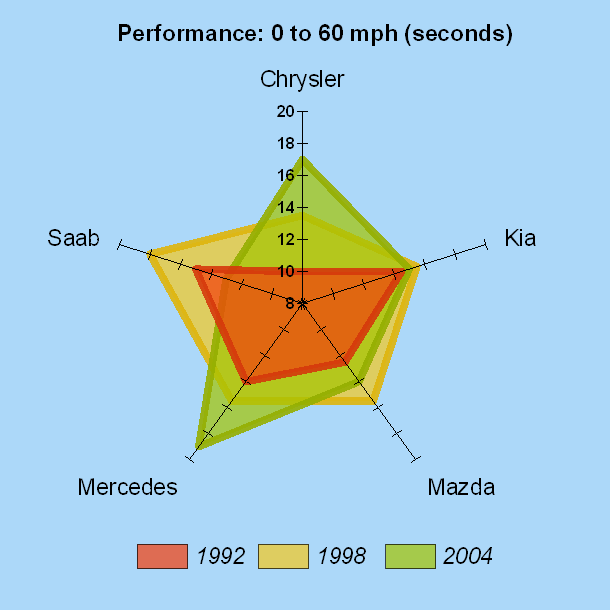Radar chart for displaying and comparing performance of several motorcars in different years. Areas enclosed by lines are filled with incremental colors, and transparency is set to the fill color of the overlay plots for comparison. Customizations for axes include the ability to set each axis to a different scale.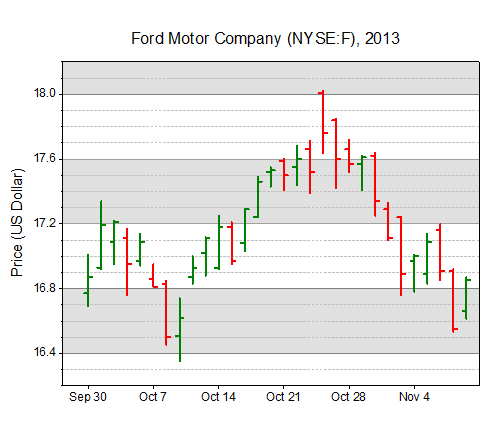Open-High-Low-Close (OHLC) chart of Ford Motor Stock Prices. OHLC chart is created from XYYYY datasets arranged in the following order: Date/Time, open price, high daily price, low daily price, closing price. Customization options include using different colors to indicate an advancing price bar (today's close higher than yesterday's) or a declining price bar.

## Profile Plots

Origin allows you to create profiles on contour and image graphs, allowing easy inspection of vertical and horizontal cross-sections of your data.

• Profile along horizontal, vertical or arbituary lines
• Multiple profiles on the same plot
• Customize profile line width and calculate profile quantities such as min, max, mean, median, sum, and SD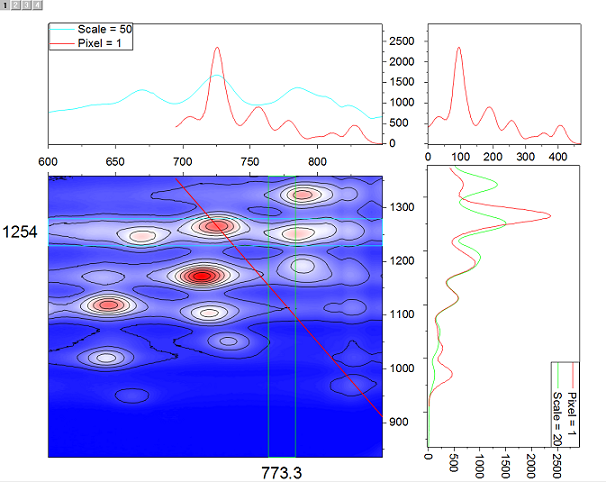Contour profile plot. The contour profiles plot provides a dynamical way to analyze the contour data and generate profiles. Multiple horizontal, vertical or arbitrary profile lines can be added on the same contour. The thickness, color and position of the line are editable. The line and projection along this line share the same color.Image graph with multiple horizontal and vertical profiles/projections. The image profiles plot provides a quick dynamical way of analyzing image data and generating profiles. Multiple horizontal, vertical or arbitrary profile lines can be added on the same image. The thickness, color and position of the line are adjustable, while the line and projection along this line share the same color.

## Function Plot

Origin supports many types of function plots, including 2D and 3D parametric functions. 2D parametric functions are widely used in describing circles, parabolas, and hyperbolas, while 3D parametric functions describe parametric surfaces.

• 2D Function Plot
• 2D Parametric Function Plot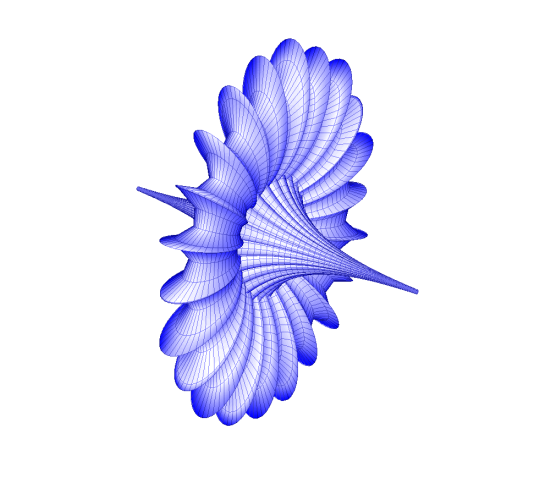A color fill surface created by a build-in 3D parametric function plot breather, which is defined by a set of formulas. Frame planes are hidden and lighting effect is turned on. More 3D Parametric Function PlotsButterfly curve created by 2D parametric function plot

X(t)=sin(t)*(exp(cos(t)))-2*cos(4*t)-(sin(t/12))^5)
Y(t)=cos(t)*(exp(cos(t)))-2*cos(4*t)-(sin(t/12))^5)
XY axes are moved to the original point.3D Parametric Function plot. All three planes and their associated axes are moved to original point, with plane grids and border turned off and directions of axes ticks changed to avoid overlapping with the surface. Lighting effect is turned on.3D Scatter plot combined with 3D Parametric Function Surfaces. Customizations include indexing symbol colors to identify different species, projecting scatter plot on XY plane, and setting transparency to the function plot surfaces so that they can be seen through.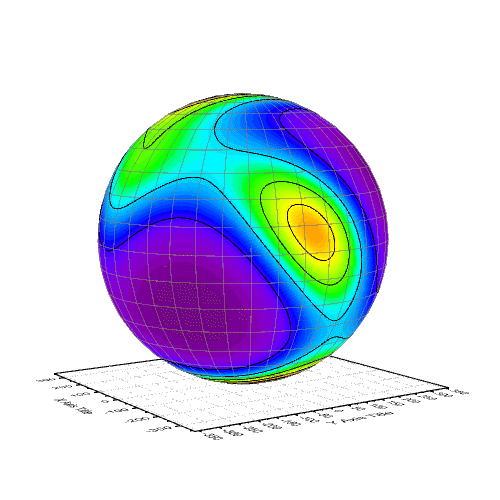Spherical contour plot of the probability distribution of the orientation of a protein domain (regulatory light chain of myosin II) in a muscle fibre. Spherical contour plot created by two 3D parametric function plots: One is a 3D colormap surface plot and another one is a 3D surface without colormap and only shows the mesh line.

## Image Graphs

Origin comes with two built-in image graph types: image plots and image profiles.Image graph with multiple horizontal and vertical profiles/projections. The image profiles plot provides a quick dynamical way of analyzing image data and generating profiles. Multiple horizontal, vertical or arbitrary profile lines can be added on the same image. The thickness, color and position of the line are adjustable, while the line and projection along this line share the same color.

 © OriginLab Corporation. All rights reserved.